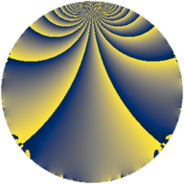# Properties

 Label 338.2.aLevel $338$ Weight $2$ Character orbit 338.a Rep. character $\chi_{338}(1,\cdot)$ Character field $\Q$ Dimension $12$ Newform subspaces $8$ Sturm bound $91$ Trace bound $3$

# Related objects

## Defining parameters

 Level: $$N$$ $$=$$ $$338 = 2 \cdot 13^{2}$$ Weight: $$k$$ $$=$$ $$2$$ Character orbit: $$[\chi]$$ $$=$$ 338.a (trivial) Character field: $$\Q$$ Newform subspaces: $$8$$ Sturm bound: $$91$$ Trace bound: $$3$$ Distinguishing $$T_p$$: $$3$$, $$5$$

## Dimensions

The following table gives the dimensions of various subspaces of $$M_{2}(\Gamma_0(338))$$.

Total New Old
Modular forms 59 12 47
Cusp forms 32 12 20
Eisenstein series 27 0 27

The following table gives the dimensions of the cuspidal new subspaces with specified eigenvalues for the Atkin-Lehner operators and the Fricke involution.

$$2$$$$13$$FrickeDim.
$$+$$$$+$$$$+$$$$2$$
$$+$$$$-$$$$-$$$$4$$
$$-$$$$+$$$$-$$$$5$$
$$-$$$$-$$$$+$$$$1$$
Plus space$$+$$$$3$$
Minus space$$-$$$$9$$

## Trace form

 $$12 q + 2 q^{3} + 12 q^{4} + 4 q^{5} + 4 q^{6} + 10 q^{9} + O(q^{10})$$ $$12 q + 2 q^{3} + 12 q^{4} + 4 q^{5} + 4 q^{6} + 10 q^{9} - 2 q^{10} - 4 q^{11} + 2 q^{12} - 4 q^{14} + 12 q^{16} + 4 q^{17} - 8 q^{18} - 8 q^{19} + 4 q^{20} + 4 q^{21} + 6 q^{22} + 4 q^{24} + 10 q^{25} + 8 q^{27} - 14 q^{29} - 12 q^{30} - 12 q^{33} - 12 q^{35} + 10 q^{36} + 4 q^{37} - 6 q^{38} - 2 q^{40} - 4 q^{42} + 2 q^{43} - 4 q^{44} + 4 q^{46} - 16 q^{47} + 2 q^{48} + 16 q^{49} + 8 q^{50} - 20 q^{51} - 18 q^{53} + 4 q^{54} + 8 q^{55} - 4 q^{56} + 16 q^{57} + 4 q^{58} + 16 q^{59} - 10 q^{61} - 16 q^{62} - 8 q^{63} + 12 q^{64} - 8 q^{66} - 12 q^{67} + 4 q^{68} - 28 q^{69} + 4 q^{70} + 8 q^{71} - 8 q^{72} + 8 q^{73} - 18 q^{74} - 38 q^{75} - 8 q^{76} - 12 q^{77} - 20 q^{79} + 4 q^{80} - 20 q^{81} - 4 q^{82} - 12 q^{83} + 4 q^{84} - 12 q^{85} + 4 q^{86} - 20 q^{87} + 6 q^{88} - 2 q^{90} + 16 q^{93} - 16 q^{94} - 12 q^{95} + 4 q^{96} - 4 q^{97} + 24 q^{99} + O(q^{100})$$

## Decomposition of $$S_{2}^{\mathrm{new}}(\Gamma_0(338))$$ into newform subspaces

Label Dim $A$ Field CM Traces A-L signs $q$-expansion
$a_{2}$ $a_{3}$ $a_{5}$ $a_{7}$ 2 13
338.2.a.a $1$ $2.699$ $$\Q$$ None $$-1$$ $$-3$$ $$1$$ $$-1$$ $+$ $+$ $$q-q^{2}-3q^{3}+q^{4}+q^{5}+3q^{6}-q^{7}+\cdots$$
338.2.a.b $1$ $2.699$ $$\Q$$ None $$-1$$ $$-1$$ $$3$$ $$3$$ $+$ $-$ $$q-q^{2}-q^{3}+q^{4}+3q^{5}+q^{6}+3q^{7}+\cdots$$
338.2.a.c $1$ $2.699$ $$\Q$$ None $$-1$$ $$0$$ $$1$$ $$-4$$ $+$ $+$ $$q-q^{2}+q^{4}+q^{5}-4q^{7}-q^{8}-3q^{9}+\cdots$$
338.2.a.d $1$ $2.699$ $$\Q$$ None $$1$$ $$-1$$ $$-3$$ $$-3$$ $-$ $-$ $$q+q^{2}-q^{3}+q^{4}-3q^{5}-q^{6}-3q^{7}+\cdots$$
338.2.a.e $1$ $2.699$ $$\Q$$ None $$1$$ $$0$$ $$-1$$ $$4$$ $-$ $+$ $$q+q^{2}+q^{4}-q^{5}+4q^{7}+q^{8}-3q^{9}+\cdots$$
338.2.a.f $1$ $2.699$ $$\Q$$ None $$1$$ $$1$$ $$3$$ $$1$$ $-$ $+$ $$q+q^{2}+q^{3}+q^{4}+3q^{5}+q^{6}+q^{7}+\cdots$$
338.2.a.g $3$ $2.699$ $$\Q(\zeta_{14})^+$$ None $$-3$$ $$3$$ $$-2$$ $$4$$ $+$ $-$ $$q-q^{2}+(1-\beta _{1}-\beta _{2})q^{3}+q^{4}-2\beta _{1}q^{5}+\cdots$$
338.2.a.h $3$ $2.699$ $$\Q(\zeta_{14})^+$$ None $$3$$ $$3$$ $$2$$ $$-4$$ $-$ $+$ $$q+q^{2}+(1-\beta _{1}-\beta _{2})q^{3}+q^{4}+2\beta _{1}q^{5}+\cdots$$

## Decomposition of $$S_{2}^{\mathrm{old}}(\Gamma_0(338))$$ into lower level spaces

$$S_{2}^{\mathrm{old}}(\Gamma_0(338)) \cong$$ $$S_{2}^{\mathrm{new}}(\Gamma_0(26))$$$$^{\oplus 2}$$$$\oplus$$$$S_{2}^{\mathrm{new}}(\Gamma_0(169))$$$$^{\oplus 2}$$